# Interpret and Stress-Test Deep Learning Networks for Probability of Default

Train a credit risk for probability of default (PD) prediction using a deep neural network. The example also shows how to use the locally interpretable model-agnostic explanations (LIME) and Shapley values interpretability techniques to understand the predictions of the model. In addition, the example analyzes model predictions for out-of-sample values and performs a stress-testing analysis.

The Stress Testing of Consumer Credit Default Probabilities Using Panel Data (Risk Management Toolbox) example presents a similar workflow but uses a logistic model. The Modeling Probabilities of Default with Cox Proportional Hazards (Risk Management Toolbox) example uses a Cox regression, or Cox proportional hazards model. However, interpretability techniques are not discussed in either of these examples because the models are simpler and interpretable. The Compare Deep Learning Networks for Credit Default Prediction example focuses on alternative network designs and fits simpler models without the macroeconomic variables.

While you can use these alternative, simpler models successfully to model credit risk, this example introduces explainability tools for exploring complex-modeling techniques in credit applications. To visualize and interpret the model predictions, you use Deep Learning Toolbox™ and the `lime` (Statistics and Machine Learning Toolbox) and `shapley` (Statistics and Machine Learning Toolbox) functions. To run this example, you:

1. Load and prepare credit data, reformat predictors, and split the data into training, validation, and testing sets.

2. Define a network architecture, select training options, and train the network. (A saved version of the trained network `residualTrainedNetworkMacro` is available for convenience.)

3. Apply the LIME and Shapley interpretability techniques on observations of interest (or "query points") to determine if the importance of predictors in the model is as expected.

4. Explore extreme predictor out-of-sample values to investigate the behavior of the model for new, extreme data.

5. Use the model to perform a stress-testing analysis of the predicted PD values.

Load the retail credit panel data set including its macroeconomic variables. The main data set (`data`) contains the following variables:

• `ID`: Loan identifier

• `ScoreGroup`: Credit score at the beginning of the loan, discretized into three groups, `High Risk`, `Medium Risk`, and `Low Risk`

• `YOB`: Years on books

• `Default`: Default indicator; the response variable

• `Year`: Calendar year

The small data set (`dataMacro`) contains macroeconomic data for the corresponding calendar years:

• `Year`: Calendar year

• `GDP`: Gross domestic product growth (year over year)

• `Market`: Market return (year over year)

The variables `YOB`, `Year`, `GDP`, and `Market` are observed at the end of the corresponding calendar year. The score group is a discretization of the original credit score when the loan started. A value of `1` for `Default` means that the loan defaulted in the corresponding calendar year.

The third data set (`dataMacroStress`) contains baseline, adverse, and severely adverse scenarios for the macroeconomic variables. This table is for the stress-testing analysis.

This example uses simulated data, but the same approach has been successfully applied to real data sets.

```load RetailCreditPanelData.mat data = join(data,dataMacro); head(data)```
``` ID ScoreGroup YOB Default Year GDP Market __ __________ ___ _______ ____ _____ ______ 1 Low Risk 1 0 1997 2.72 7.61 1 Low Risk 2 0 1998 3.57 26.24 1 Low Risk 3 0 1999 2.86 18.1 1 Low Risk 4 0 2000 2.43 3.19 1 Low Risk 5 0 2001 1.26 -10.51 1 Low Risk 6 0 2002 -0.59 -22.95 1 Low Risk 7 0 2003 0.63 2.78 1 Low Risk 8 0 2004 1.85 9.48 ```

### Encode Categorical Variables

To train a deep learning network, you must first encode the categorical `ScoreGroup` variable to one-hot encoded vectors.

View the order of the `ScoreGroup` categories.

`categories(data.ScoreGroup)'`
```ans = 1×3 cell {'High Risk'} {'Medium Risk'} {'Low Risk'} ```

`ans = ``1×3 cell`

` {'High Risk'} {'Medium Risk'} {'Low Risk'}`

One-hot encode the `ScoreGroup` variable.

`riskGroup = onehotencode(data.ScoreGroup,2);`

Add the one-hot vectors to the table.

```data.HighRisk = riskGroup(:,1); data.MediumRisk = riskGroup(:,2); data.LowRisk = riskGroup(:,3);```

Remove the original `ScoreGroup` variable from the table using `removevars`.

`data = removevars(data,{'ScoreGroup'});`

Move the `Default` variable to the end of the table, as this variable is the response you want to predict.

`data = movevars(data,'Default','After','LowRisk');`

View the first few rows of the table. The `ScoreGroup` variable is split into multiple columns with the categorical values as the variable names.

`head(data)`
``` ID YOB Year GDP Market HighRisk MediumRisk LowRisk Default __ ___ ____ _____ ______ ________ __________ _______ _______ 1 1 1997 2.72 7.61 0 0 1 0 1 2 1998 3.57 26.24 0 0 1 0 1 3 1999 2.86 18.1 0 0 1 0 1 4 2000 2.43 3.19 0 0 1 0 1 5 2001 1.26 -10.51 0 0 1 0 1 6 2002 -0.59 -22.95 0 0 1 0 1 7 2003 0.63 2.78 0 0 1 0 1 8 2004 1.85 9.48 0 0 1 0 ```

### Split Data

Partition the data set into training, validation, and test partitions using the unique loan ID numbers. Set aside 60% of the data for training, 20% for validation, and 20% for testing.

Find the unique loan IDs.

```idx = unique(data.ID); numObservations = length(idx);```

Determine the number of observations for each partition.

```numObservationsTrain = floor(0.6*numObservations); numObservationsValidation = floor(0.2*numObservations); numObservationsTest = numObservations - numObservationsTrain - numObservationsValidation;```

Create an array of random indices corresponding to the observations and partition it using the partition sizes.

```rng('default'); % for reproducibility idxShuffle = idx(randperm(numObservations)); idxTrain = idxShuffle(1:numObservationsTrain); idxValidation = idxShuffle(numObservationsTrain+1:numObservationsTrain+numObservationsValidation); idxTest = idxShuffle(numObservationsTrain+numObservationsValidation+1:end);```

Find the table entries corresponding to the data set partitions.

```idxTrainTbl = ismember(data.ID,idxTrain); idxValidationTbl = ismember(data.ID,idxValidation); idxTestTbl = ismember(data.ID,idxTest);```

Keep the variables of interest for the task (`YOB`, `Default`, and `ScoreGroup`) and remove all other variables from the table.

```data = removevars(data,{'ID','Year'}); head(data)```
``` YOB GDP Market HighRisk MediumRisk LowRisk Default ___ _____ ______ ________ __________ _______ _______ 1 2.72 7.61 0 0 1 0 2 3.57 26.24 0 0 1 0 3 2.86 18.1 0 0 1 0 4 2.43 3.19 0 0 1 0 5 1.26 -10.51 0 0 1 0 6 -0.59 -22.95 0 0 1 0 7 0.63 2.78 0 0 1 0 8 1.85 9.48 0 0 1 0 ```

Partition the table of data into training, validation, and testing partitions using the indices.

```tblTrain = data(idxTrainTbl,:); tblValidation = data(idxValidationTbl,:); tblTest = data(idxTestTbl,:);```

### Define Network Architecture

You can use different deep learning architectures for the task of predicting credit default probabilities. Smaller networks are quick to train, but deeper networks can learn more abstract features. Choosing a neural network architecture requires balancing computation time against accuracy. This example uses a residual architecture. For an example of other networks, see the Compare Deep Learning Networks for Credit Default Prediction example.

Create a residual architecture (ResNet) from multiple stacks of fully connected layers and ReLU activations. ResNet architectures are state of the art in deep learning applications and popular in deep learning literature. Originally developed for image classification, ResNets have proven successful across many domains .

```residualLayers = [ featureInputLayer(6, 'Normalization', 'zscore', 'Name', 'input') fullyConnectedLayer(16, 'Name', 'fc1','WeightsInitializer','he') batchNormalizationLayer('Name', 'bn1') reluLayer('Name','relu1') fullyConnectedLayer(32, 'Name', 'resblock1-fc1','WeightsInitializer','he') batchNormalizationLayer('Name', 'resblock1-bn1') reluLayer('Name', 'resblock1-relu1') fullyConnectedLayer(32, 'Name', 'resblock1-fc2','WeightsInitializer','he') additionLayer(2, 'Name', 'resblock1-add') batchNormalizationLayer('Name', 'resblock1-bn2') reluLayer('Name', 'resblock1-relu2') fullyConnectedLayer(64, 'Name', 'resblock2-fc1','WeightsInitializer','he') batchNormalizationLayer('Name', 'resblock2-bn1') reluLayer('Name', 'resblock2-relu1') fullyConnectedLayer(64, 'Name', 'resblock2-fc2','WeightsInitializer','he') additionLayer(2, 'Name', 'resblock2-add') batchNormalizationLayer('Name', 'resblock2-bn2') reluLayer('Name', 'resblock2-relu2') fullyConnectedLayer(1, 'Name', 'fc2','WeightsInitializer','he') sigmoidLayer('Name', 'sigmoid') BinaryCrossEntropyLossLayer('output')]; residualLayers = layerGraph(residualLayers); residualLayers = addLayers(residualLayers,fullyConnectedLayer(32, 'Name', 'resblock1-fc-shortcut')); residualLayers = addLayers(residualLayers,fullyConnectedLayer(64, 'Name', 'resblock2-fc-shortcut')); residualLayers = connectLayers(residualLayers, 'relu1', 'resblock1-fc-shortcut'); residualLayers = connectLayers(residualLayers, 'resblock1-fc-shortcut', 'resblock1-add/in2'); residualLayers = connectLayers(residualLayers, 'resblock1-relu2', 'resblock2-fc-shortcut'); residualLayers = connectLayers(residualLayers, 'resblock2-fc-shortcut', 'resblock2-add/in2'); ```

You can visualize the network using Deep Network Designer or the `analyzeNetwork` function.

`deepNetworkDesigner(residualLayers)`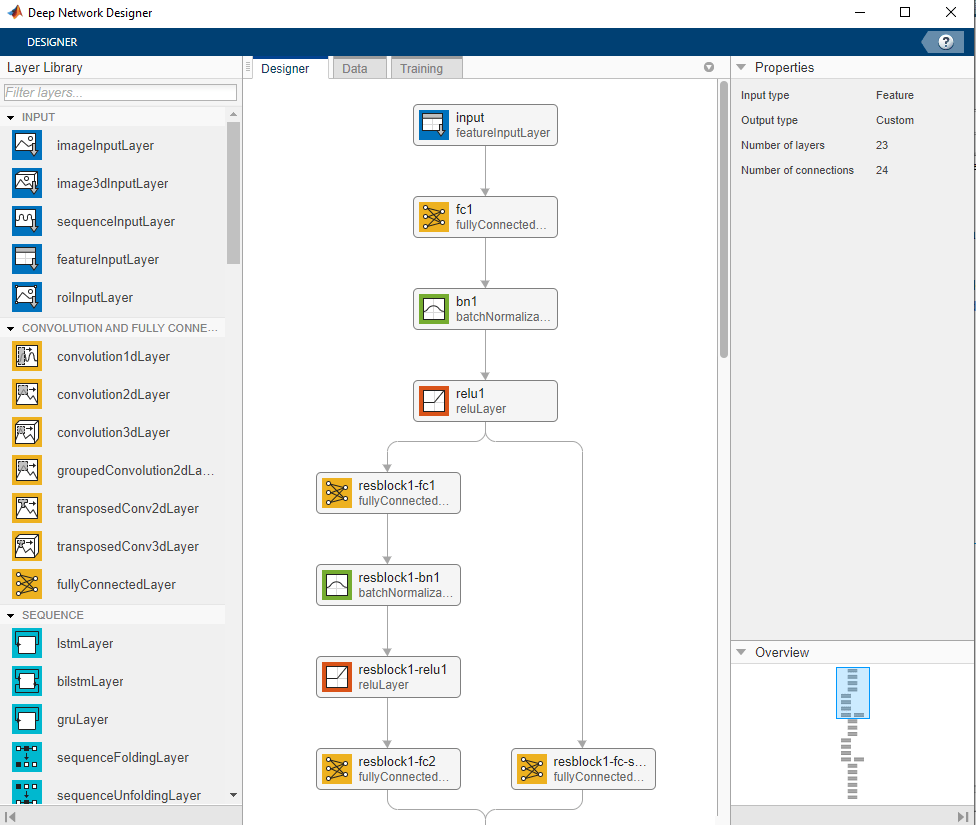### Specify Training Options

In this example, train each network with these training options:

• Train using the Adam optimizer.

• Set the initial learning rate to `0.001`.

• Set the mini-batch size to `512`.

• Train for `75` epochs.

• Turn on the training progress plot and turn off the command window output.

• Shuffle the data at the beginning of each epoch.

• Monitor the network accuracy during training by specifying validation data and using it to validate the network every 1000 iterations.

```options = trainingOptions('adam', ... 'InitialLearnRate',0.001, ... 'MiniBatchSize',512, ... 'MaxEpochs',75, ... 'Plots','training-progress', ... 'Verbose',false, ... 'Shuffle','every-epoch', ... 'ValidationData',tblValidation, ... 'ValidationFrequency',1000);```

The Compare Deep Learning Networks for Credit Default Prediction example fits the same type of network, but it excludes the macroeconomic predictors. In that example, if you increase the number of epochs from `50` to `75`, you can improve accuracy without overfitting concerns.

You can perform optimization programmatically or interactively using Experiment Manager. For an example showing how to perform a hyperparameter sweep of the training options, see Create a Deep Learning Experiment for Classification.

### Train Network

Train the network using the architecture that you defined, the training data, and the training options. By default, `trainNetwork` uses a GPU if one is available; otherwise, it uses a CPU. Training on a GPU requires Parallel Computing Toolbox™ and a supported GPU device. For information, see Deep Learning with MATLAB on Multiple GPUs. You can also specify the execution environment by using the `'ExecutionEnvironment'` name-value argument of `trainingOptions`.

To avoid waiting for the training, load pretrained networks by setting the `doTrain` flag to `false`. To train the networks using `analyzeNetwork`, set the `doTrain` flag to `true`. The Training Progress window displays progress. The training time using an NVIDIA® GeForce® RTX 2080 is about 35 minutes for 75 epochs.

```doTrain = false; if doTrain residualNetMacro = trainNetwork(tblTrain,'Default',residualLayers,options); else load residualTrainedNetworkMacro.mat end```### Test Network

Use the `predict` function to predict the default probability of the test data using the trained networks.

`tblTest.residualPred = predict(residualNetMacro,tblTest(:,1:end-1));`

Plot Default Rates by Year on Books

To assess the performance of the network, use the `groupsummary` function to group the true default rates and corresponding predictions by years on the books (represented by the `YOB` variable) and calculate the mean value.

```summaryYOB = groupsummary(tblTest,'YOB','mean',{'Default','residualPred'}); head(summaryYOB)```
``` YOB GroupCount mean_Default mean_residualPred ___ __________ ____________ _________________ 1 19364 0.017352 0.017688 2 18917 0.012158 0.013354 3 18526 0.011875 0.011522 4 18232 0.011683 0.010485 5 17925 0.0082008 0.0090247 6 17727 0.0066565 0.0066525 7 12294 0.0030909 0.0034051 8 6361 0.0017293 0.0018151 ```

Plot the true average default rate against the average predictions by `YOB`.

```figure scatter(summaryYOB.YOB,summaryYOB.mean_Default*100,'*'); hold on plot(summaryYOB.YOB,summaryYOB.mean_residualPred*100); hold off title('Residual Network') xlabel('Years on Books') ylabel('Default Rate (%)') legend('Observed','Predicted')```The plot shows a good fit on the test data. The model seems to capture the overall trend as the age of the loan (`YOB` value) increases, as well as changes in the steepness of the trend.

The rest of this example shows some ways to better understand the model. First, it reviews standard explainability techniques that you can apply to this model, specifically, the `lime` (Statistics and Machine Learning Toolbox) and `shapley` (Statistics and Machine Learning Toolbox) functions. Then, it explores the behavior of the model in new (out-of-sample) data values. Finally, the example uses the model to predict PD values under stressed macroeconomic conditions, also known as stress testing.

### Explain Model with LIME and Shapley

The local interpretable model-agnostic explanations (LIME) method and the Shapley method both aim to explain the behavior of the model at a particular observation of interest or "query point." More specifically, these techniques help you to understand the importance of each variable in the prediction made for a particular observation. For more information, see `lime` (Statistics and Machine Learning Toolbox) and `shapley` (Statistics and Machine Learning Toolbox).

For illustration purposes, choose two observations from the data to better interpret the model predictions. The response values (last column) are not needed.

The first observation is a seasoned, low-risk loan. In other words, it has an initial score of `LowRisk` and eight years on the books.

```obs1 = data(8,1:end-1); disp(obs1)```
``` YOB GDP Market HighRisk MediumRisk LowRisk ___ ____ ______ ________ __________ _______ 8 1.85 9.48 0 0 1 ```

The second observation is a new, high-risk loan. That is, the score is `HighRisk` and it is in its first year on the books.

```obs2 = data(88,1:end-1); disp(obs2)```
``` YOB GDP Market HighRisk MediumRisk LowRisk ___ ____ ______ ________ __________ _______ 1 2.72 7.61 1 0 0 ```

Both `lime` (Statistics and Machine Learning Toolbox) and `shapley` (Statistics and Machine Learning Toolbox) require a reference data set with predictor values. This reference data can be the training data itself, or any other reference data where the model can be evaluated to explore the behavior of the model. More data points allow the explainability methods to understand the behavior of the model in more regions. However, a large data set can also slow down the computations, especially for `shapley` (Statistics and Machine Learning Toolbox). For illustration purposes, use the first 1000 rows from the training data set. The response values (last column) are not needed.

`predictorData = data(1:1000,1:end-1);`

`lime` (Statistics and Machine Learning Toolbox) and `shapley` (Statistics and Machine Learning Toolbox) also require a function handle to the `predict` function. Treat `predict` like a black-box model and call it multiple times to make predictions on data and gather information on the behavior of the model.

`blackboxFcn = @(x)predict(residualNetMacro,x);`

#### Create `lime` Object

Create a `lime` (Statistics and Machine Learning Toolbox) object by passing the black-box function handle and the selected predictor data.

Randomly generated synthetic data underlying `lime` (Statistics and Machine Learning Toolbox) can affect the importance. The report may change depending on the synthetic data generated. It can also change due to optional arguments, such as the `'KernelWidth'` parameter that controls the area around the observation of interest ("query point") while you fit the local model.

`explainerLIME = lime(blackboxFcn,predictorData,'Type','regression');`

Choose a number of important predictors of interest and fit a local model around the selected observations. For illustration purposes, the model contains all of the predictors.

```numImportantPredictors = 6; explainerObs1 = fit(explainerLIME,obs1,numImportantPredictors); explainerObs2 = fit(explainerLIME,obs2,numImportantPredictors);```

Plot the importance for each predictor.

```figure subplot(2,1,1) plot(explainerObs1); subplot(2,1,2) plot(explainerObs2);```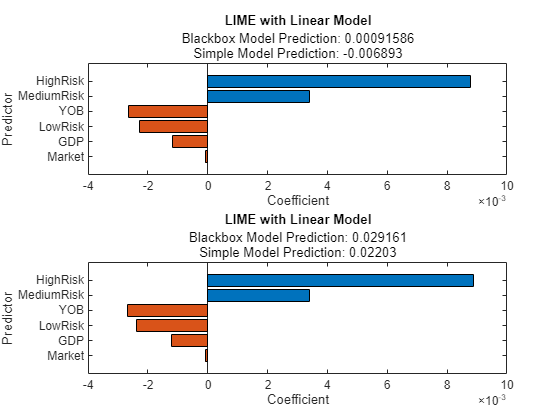The `lime` (Statistics and Machine Learning Toolbox) results are quite similar for both observations. The information in the plots show that the most important variables are the `High` `Risk` and `Medium` `Risk` variables. `High` `Risk` and `Medium` `Risk` contribute positively to higher probabilities of default. On the other hand, `YOB,` `LowRisk`, `GDP`, and `Market` have a negative contribution to the default probability. The `Market` variable does not seem to contribute as much as the other variables. The values in the plots are coefficients of a simple model fitted around the point of interest, so the values can be interpreted as sensitivities of the PD to the different predictors, and these results seem to align with expectations. For example, PD predictions decrease as the `YOB` value (age of the loan) increases, consistent with the downward trend observed in the model fit plot in the Test Network section.

#### Create `shapley` Object

The steps for creating a `shapley` (Statistics and Machine Learning Toolbox) object are the same as for `lime` (Statistics and Machine Learning Toolbox). Create a `shapley` (Statistics and Machine Learning Toolbox) object by passing the black-box function handle and the predictor data selected previously.

The `shapley` (Statistics and Machine Learning Toolbox) analysis can also be affected by randomly generated data, and it requires different methods to control the simulations required for the analysis. For illustration purposes, create the `shapley` (Statistics and Machine Learning Toolbox) object with default settings.

`explainerShapley = shapley(blackboxFcn,predictorData);`

Find and plot the importance of predictors for each query point. `shapley` (Statistics and Machine Learning Toolbox) is more computationally intensive than `lime` (Statistics and Machine Learning Toolbox). As the number of rows in the predictor data increases, the computational time for the `shapley` (Statistics and Machine Learning Toolbox) results increases. For large data sets, using parallel computing is recommended (see the `'UseParallel'` option in `shapley` (Statistics and Machine Learning Toolbox)).

```explainerShapleyObs1 = fit(explainerShapley, obs1); explainerShapleyObs2 = fit(explainerShapley, obs2); figure; subplot(2,1,1) plot(explainerShapleyObs1) subplot(2,1,2) plot(explainerShapleyObs2)```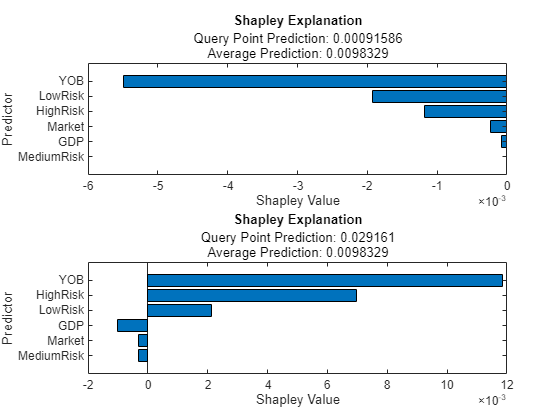In this case, the results look different for the two observations. The `shapley` (Statistics and Machine Learning Toolbox) results explain the deviations from the average PD prediction. For the first observation, which is a very low risk observation, the predicted value is well below the average PD Therefore, all `shapley` (Statistics and Machine Learning Toolbox) values are negative, with `YOB` being the most important variable in this case, followed by `LowRisk`. For the second observation, which is a very high risk observation, most `shapley` (Statistics and Machine Learning Toolbox) values are positive, with `YOB` and `HighRisk` as the main contributors to a predicted PD well above average.

### Explore Out-of-Sample Model Predictions

Splitting the original data set into training, validation, and testing helps prevent overfitting. However, the validation and test data sets share similar characteristics with the training data, for example, the range of values for `YOB`, or the observed values for the macroeconomic variables.

`rangeYOB = [min(data.YOB) max(data.YOB)]`
```rangeYOB = 1×2 1 8 ```
`rangeGDP = [min(data.GDP) max(data.GDP)]`
```rangeGDP = 1×2 -0.5900 3.5700 ```
`rangeMarket = [min(data.Market) max(data.Market)]`
```rangeMarket = 1×2 -22.9500 26.2400 ```

You can explore the behavior of the out-of-sample (OOS) model in two different ways. First, you can predict for age values (`YOB` variable) larger than the maximum age value observed in the data. You can predict `YOB` values up to `15`. Second, you can predict for economic conditions not observed in the data either. This example uses two extremely severe macroeconomic situations, where both the `GDP` and `Market` values are very negative and outside the range of values in the data.

Start by setting up a baseline scenario where the last macroeconomic data in the sample is used as reference. The `YOB` values go out of sample for all scenarios.

```dataBaseline = table; dataBaseline.YOB = repmat((1:15)',3,1); dataBaseline.GDP = zeros(size(dataBaseline.YOB)); dataBaseline.Market = zeros(size(dataBaseline.YOB)); dataBaseline.HighRisk = zeros(size(dataBaseline.YOB)); dataBaseline.MediumRisk = zeros(size(dataBaseline.YOB)); dataBaseline.LowRisk = zeros(size(dataBaseline.YOB)); dataBaseline.GDP(:) = data.GDP(8); dataBaseline.Market(:) = data.Market(8); dataBaseline.HighRisk(1:15) = 1; dataBaseline.MediumRisk(16:30) = 1; dataBaseline.LowRisk(31:45) = 1; disp(head(dataBaseline))```
``` YOB GDP Market HighRisk MediumRisk LowRisk ___ ____ ______ ________ __________ _______ 1 1.85 9.48 1 0 0 2 1.85 9.48 1 0 0 3 1.85 9.48 1 0 0 4 1.85 9.48 1 0 0 5 1.85 9.48 1 0 0 6 1.85 9.48 1 0 0 7 1.85 9.48 1 0 0 8 1.85 9.48 1 0 0 ```

Create two new extreme scenarios that include out-of-sample values not only for `YOB`, but also for the macroeconomic variables. This example uses pessimistic scenarios, but you could repeat the analysis for optimistic situations to explore the behavior of the model in either kind of extreme situation.

```dataExtremeS1 = dataBaseline; dataExtremeS1.GDP(:) = -1; dataExtremeS1.Market(:) = -25; dataExtremeS2 = dataBaseline; dataExtremeS2.GDP(:) = -2; dataExtremeS2.Market(:) = -40;```

Predict PD values for all scenarios using `predict`.

```dataBaseline.PD = predict(residualNetMacro,dataBaseline); dataExtremeS1.PD = predict(residualNetMacro,dataExtremeS1); dataExtremeS2.PD = predict(residualNetMacro,dataExtremeS2);```

Visualize the results for a selected score. For convenience, the average of the PD values over the three scores is visualized as a summary.

```ScoreSelected ="High"; switch ScoreSelected case 'High' ScoreInd = dataBaseline.HighRisk==1; PredPDYOB = [dataBaseline.PD(ScoreInd) dataExtremeS1.PD(ScoreInd) dataExtremeS2.PD(ScoreInd)]; case 'Medium' ScoreInd = dataBaseline.MediumRisk==1; PredPDYOB = [dataBaseline.PD(ScoreInd) dataExtremeS1.PD(ScoreInd) dataExtremeS2.PD(ScoreInd)]; case 'Low' ScoreInd = dataBaseline.LowRisk==1; PredPDYOB = [dataBaseline.PD(ScoreInd) dataExtremeS1.PD(ScoreInd) dataExtremeS2.PD(ScoreInd)]; case 'Average' PredPDYOBBase = groupsummary(dataBaseline,'YOB','mean','PD'); PredPDYOBS1 = groupsummary(dataExtremeS1,'YOB','mean','PD'); PredPDYOBS2 = groupsummary(dataExtremeS2,'YOB','mean','PD'); PredPDYOB = [PredPDYOBBase.mean_PD PredPDYOBS1.mean_PD PredPDYOBS2.mean_PD]; end figure; bar(PredPDYOB*100); xlabel('Years on Books') ylabel('Probability of Default (%)') legend('Baseline','Scenario 1','Scenario 2') title(strcat("Out-of-Sample Scenarios, ",ScoreSelected," Score")) grid on```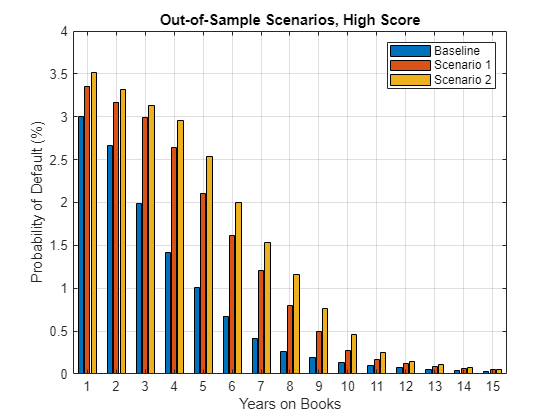The overall results are in line with expectations, since the PD values decrease as the `YOB` value increases, and worse economic conditions result in higher PD values. However, the relative increase of the predicted PD values shows an interesting result. For `Low` and `Medium` scores, there is a significant increase for the first year on books (`YOB` = `1`). In contrast, for `High` scores, the relative increase from baseline, to the first extreme scenario, then to the second extreme case, is small. This result suggests an implicit upper limit in the predicted values in the structure of the model. The extreme scenarios in this exercise seem unlikely to occur, however, for extreme but plausible scenarios, this behavior would require investigation with stress testing.

### Stress-Test Predicted Probabilities of Default (PD)

Because the model includes macroeconomic variables, it can be used to perform a stress-testing analysis (see for example , , ). The steps are similar to the previous section except that the scenarios are plausible scenarios set periodically at an institution level, or set by regulators to be used by all institutions.

The `dataMacroStress` data set contains three scenarios for the stress testing of the model, namely, baseline, adverse, and severely adverse scenarios. The adverse and severe scenarios are relative to the baseline scenario, and the macroeconomic conditions are plausible given the baseline. These scenarios fall within the range of values observed in the data used for training and validation. The stress testing of the PD values for given macroeconomic scenarios is conceptually different from the exercise in the previous section, where the focus is on exploring the behavior of the model on out-of-sample data, regardless of how plausible those extreme scenarios are from an economic point of view.

Following the prior steps, you generate PD predictions for each score level and each scenario.

```dataBaselineStress = dataBaseline(:,1:end-1); dataAdverse = dataBaselineStress; dataSevere = dataBaselineStress; dataBaselineStress.GDP(:) = dataMacroStress{'Baseline','GDP'}; dataBaselineStress.Market(:) = dataMacroStress{'Baseline','Market'}; dataAdverse.GDP(:) = dataMacroStress{'Adverse','GDP'}; dataAdverse.Market(:) = dataMacroStress{'Adverse','Market'}; dataSevere.GDP(:) = dataMacroStress{'Severe','GDP'}; dataSevere.Market(:) = dataMacroStress{'Severe','Market'}; ```

Use the `predict` function to predict PD values for all scenarios. Visualize the results for a selected score.

```dataBaselineStress.PD = predict(residualNetMacro,dataBaselineStress); dataAdverse.PD = predict(residualNetMacro,dataAdverse); dataSevere.PD = predict(residualNetMacro,dataSevere); ScoreSelected =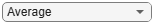"Average"; switch ScoreSelected case 'High' ScoreInd = dataBaselineStress.HighRisk==1; PredPDYOBStress = [dataBaselineStress.PD(ScoreInd) dataAdverse.PD(ScoreInd) dataSevere.PD(ScoreInd)]; case 'Medium' ScoreInd = dataBaselineStress.MediumRisk==1; PredPDYOBStress = [dataBaselineStress.PD(ScoreInd) dataAdverse.PD(ScoreInd) dataSevere.PD(ScoreInd)]; case 'Low' ScoreInd = dataBaselineStress.LowRisk==1; PredPDYOBStress = [dataBaselineStress.PD(ScoreInd) dataAdverse.PD(ScoreInd) dataSevere.PD(ScoreInd)]; case 'Average' PredPDYOBBaseStress = groupsummary(dataBaselineStress,'YOB','mean','PD'); PredPDYOBAdverse = groupsummary(dataAdverse,'YOB','mean','PD'); PredPDYOBSevere = groupsummary(dataSevere,'YOB','mean','PD'); PredPDYOBStress = [PredPDYOBBaseStress.mean_PD PredPDYOBAdverse.mean_PD PredPDYOBSevere.mean_PD]; end figure; bar(PredPDYOBStress*100); xlabel('Years on Books') ylabel('Probability of Default (%)') legend('Baseline','Adverse','Severe') title(strcat("PD Stress Testing, ",ScoreSelected," Score")) grid on```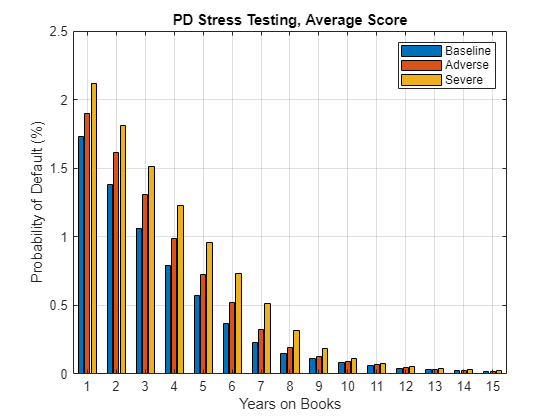The overall results are in line with expectations. As in the Explore Out-of-Sample Model Predictions section, the predictions for the `High` score in the first year on books (`YOB` = `1`) needs to be reviewed, since the relative increase in the predicted PD from one scenario to the next seems smaller than for other scores and loan ages. All other predictions show a reasonable pattern that are consistent with expectations.

### References

 He, Kaiming, Xiangyu Zhang, Shaoqing Ren, and Jian Sun. "Deep residual learning for image recognition." In Proceedings of the IEEE Conference on Computer Vision and Pattern Recognition. pp. 770–778, 2016.

 Federal Reserve, Comprehensive Capital Analysis and Review (CCAR): https://www.federalreserve.gov/bankinforeg/ccar.htm

 Bank of England, Stress Testing: https://www.bankofengland.co.uk/financial-stability

 European Banking Authority, EU-Wide Stress Testing: https://www.eba.europa.eu/risk-analysis-and-data/eu-wide-stress-testing